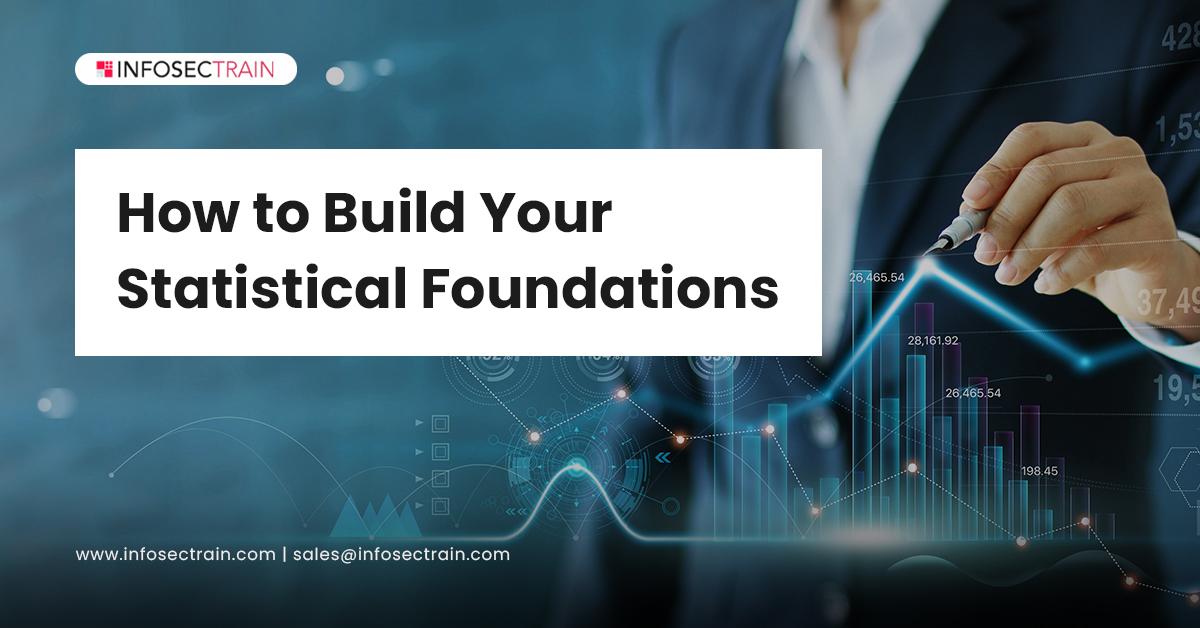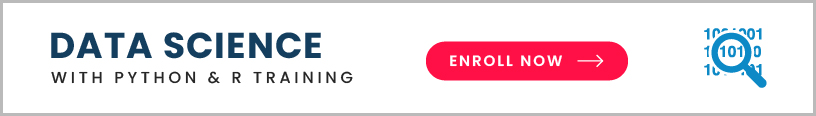Upgrade Your Career with Exciting Offers on our Career-defining Courses Upto 50% OFF | Offer ending in:
D H M S Grab Now
X

# How to Build Your Statistical Foundations for a Career in Data Science?

Data science is a field that spans many disciplines. It is not merely in control of the digital world. It is used for everything from internet searches to social media feeds to political campaigns, grocery store inventory, airline routes, and medical appointments. A Data Scientist should acquire a complete set of abilities that covers each building block of the discipline in order to have a successful career. Statistics is one of the building blocks.Having statistical knowledge will help you better use data insights while you are just starting in data science. This article will explore how you can build your statistical foundations for a career in data science.

Overview of statistics:

Let’s start with a description of the term statistics. Statistics is a branch of mathematics concerned with data collection, presentation, analysis, and interpretation. It is commonly used to comprehend complex real-world problems and simplify them to make well-informed judgments. To assess primary data, develop a statistical model, and forecast outcomes, a variety of statistical principles, functions, and algorithms can be applied. Any scenario can be examined in one of two ways:

• Statistical analysis: Statistical analysis or quantitative analysis is the science of identifying patterns and trends by collecting, exploring, and presenting massive amounts of data.
• Non-statistical analysis: Non-statistical or qualitative analysis encompasses text, sound, still images, and moving images and provides generic information.

While both types of analysis produce results, the statistical analysis provides more significant insights and a better picture, essential for organizations.

Types of statistics:

Statistics are divided into two categories.

1. Descriptive statistics: It assists in the organization of data and focuses on the data’s most important properties. It presents a numerical or graphical overview of the data. Numerical metrics such as average, mode, standard deviation, or SD, as well as correlation, are used to explain the data set’s characteristics.
2. Inferential statistics: It uses probability theory to generalize the more extensive data set. It allows you to model relationships within the data and deduce population parameters based on sample statistics. You can use modeling to create mathematical equations that describe the relationships between two or more variables.

The field of statistics has an impact on our lives in a variety of ways, from our daily life at home to the business of running the world’s largest cities. Statistics have an impact on everything. When dealing with statistics, there are a number of statistical terms to be aware of:

• Population: The group from which data is to be collected is a population.
• Sample: A subset of a population is referred to as a sample.
• Variable: A variable is a feature that distinguishes one member of the population from another in terms of quality or quantity.
• Quantitative variable: A variable differing in quantity.
• Qualitative variable: A variable differing in quality.
• Discrete variable: It is one in which no value can be assumed between two given values.
• Continuous variable: It is one in which any value can be considered between the two given values.Importance of statistics in data science

Data is ingrained in today’s world; individuals and businesses generate vast amounts of data that professionals can only view and comprehend. While a career in data science may appear appealing and accessible, aspiring Data Scientists should assess their familiarity with statistics before making their next move. Statistics provides the techniques and tools for discovering structure in large datasets and provides individuals and organizations with a better awareness of the realities revealed by their data.

Vital statistical concepts for data science:

The core ideas of descriptive statistics and probability theory, which include the key concepts of probability distribution, statistical significance, hypothesis testing, regression, and Bayesian thinking, are essential for Data Scientists to comprehend.

General statistical concepts: Bias, variance, mean, median, mode, and percentiles are some of the most fundamental concepts in statistics. Understanding data kinds (rectangular and non-rectangular), location estimation, variability estimation, data distributions, binary and categorical data, correlation, and relationships between different types of factors.

Probability theory: Probability is a branch of mathematics that calculates the chances of a random event occurring. A random experiment is a physical setting with an unknown outcome until it is observed. The greater the likelihood, the closer to one, the more likely it is that it will occur.

Probability distribution: All possible outcomes of the random variable are represented by a probability distribution. Data Scientists use them to determine how likely specific numbers or events are. Expected value, variance, skewness, and kurtosis are among them. The standard deviation is equal to the square root of the variance.

Bayesian statistics: Bayesian thinking entails revising beliefs in light of new information. This is an alternative to the regularly used frequency statistics for calculating probabilities. The probability of an event is determined utilizing frequency statistics, which leverages existing data. Bayesian statistics take into consideration factors that we expect to be true in the future. For example, you can predict whether at least 100 people will visit your coffee shop every Saturday for the next year.

Over and under-sampling: Not all data sets are the same; therefore, Data Scientists utilize over-sampling and under-sampling to change unequal data sets, often known as resampling. Synthetic Minority Over-Sampling Technique (SMOTE) is one of the well-known approaches for imitating a naturally occurring sample.

Dimension reduction: Data Scientists can limit the number of random variables under consideration using feature selection and feature extraction. This streamlines the process of entering data into algorithms and simplifies data models.

Regression: In simple terms, regression determines a link between the independent and dependent variables. Regression can be divided into two types: linear regression and multilinear regression.

Experiments in statistics and significance testing: Testing gives identifying the circumstances in which the action should be taken or not, based on what results it will produce. There are other tests as well like A/B Testing, Z Test, T-Test, Null Hypothesis with similar relevance to science, resampling, statistical significance, confidence interval, p-value, alpha, degree of freedom, ANOVA, critical values, covariance and correlation, effect size, statistical power.

Best way to learn statistics for data science:

Matching your needs with the most appropriate training resources is crucial to getting the greatest data science education. For example, based on a person’s educational and professional experience, the process of learning statistics in data science will seem different.

Three prominent educational paths are:

1. Massive Open Online Courses (MOOCs)
2. Bootcamps
3. Master’s programs